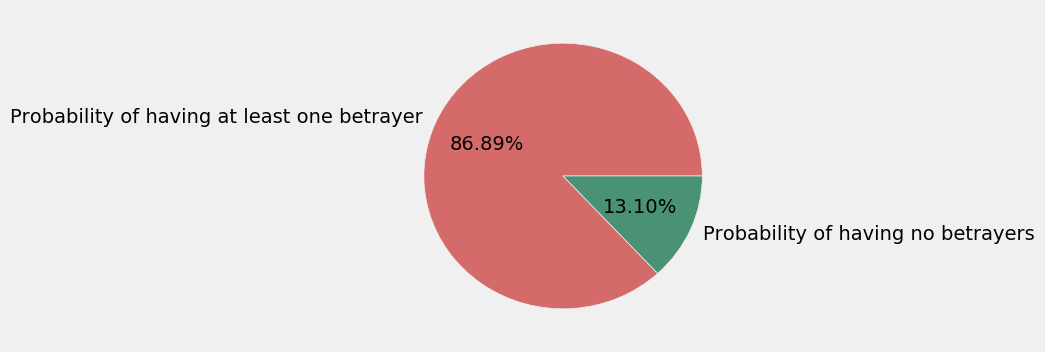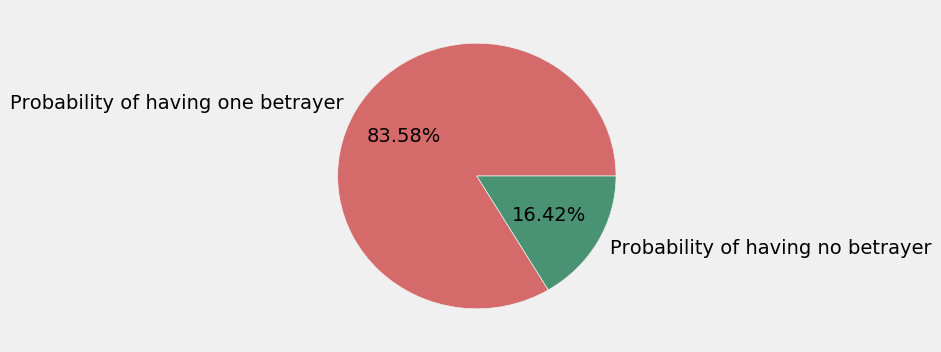# Simulation of the Official and Alternative processes of dealing secret role cards in Dead of Winter¶

Here we run code that simulates both the official and alternative process of dealing secret role cards. We are using a uniform random sample

In :
%matplotlib inline
import matplotlib.pyplot as plt
import numpy as np
import pandas as pd

plt.style.use('fivethirtyeight')
m = 100000


# Official Process Simulation¶

## The official distribution scheme for secret role cards¶

1. Separate the secret role cards into a betrayer and non-betrayer pile
2. For each player, do the following
1. Get two cards from the non-betrayer pile randomly face-down
2. Get one card from the betrayer pile face-down
3. Shuffle the cards into one pile
4. Let the play pick one random
In :
def get_number_of_betrayers_official_df(number_of_iterations):
results = []
for _ in range(number_of_iterations):
players_choice = []
players_choice.append(np.random.choice([1, 0, 0], 5))
results.append(np.sum(players_choice))
return pd.DataFrame(results, columns=['number_of_betrayers'])

In :
nofbo = get_number_of_betrayers_official_df(m)

Out:
number_of_betrayers
0 1
1 3
2 1
3 0
4 1
In :
def has_at_least_one_betrayer(row):
if row['number_of_betrayers'] > 0:
return 1
else:
return 0

# Add a new column whose values are a flag if there are betrayers or not in a game
nofbo['has_betrayers'] = nofbo.apply(lambda row: has_at_least_one_betrayer(row), axis=1)

Out:
number_of_betrayers has_betrayers
0 1 1
1 3 1
2 1 1
3 0 0
4 1 1
In :
percentage_betrayers = (float(nofbo['has_betrayers'].sum()) / nofbo.shape) * 100
percentage_betrayers

Out:
86.895
In :
percentage_non_betrayers = 100 - percentage_betrayers
percentage_non_betrayers

Out:
13.105000000000004
In :
plt.figure(figsize=(4,4))
plt.pie([percentage_betrayers, percentage_non_betrayers],
labels=['Probability of having at least one betrayer', 'Probability of having no betrayers'],
autopct='%.2f%%',
colors=['#D46A6A', '#499273'])

Out:
([<matplotlib.patches.Wedge at 0x10bf3a550>,
<matplotlib.patches.Wedge at 0x10bf44780>],
<matplotlib.text.Text at 0x10bf44f60>],
[<matplotlib.text.Text at 0x10bf44240>,
<matplotlib.text.Text at 0x10bf4b438>])# Alternative Process Simulation¶

## The alternative distribution scheme for secret role cards¶

1. Separate the secre role cards into a betrayer and non-betrayer pile
2. Pick 5 cards from the non-betrayer pile and 1 card from the betrayer pile randomly
3. Deal 1 card randomly to each player
In :
def get_number_of_betrayers_alternative_df(number_of_iterations):
cards = [1, 0, 0, 0, 0, 0]
results = []
for _ in range(number_of_iterations):
results.append(np.sum(np.random.choice(cards, 5, replace=False)))
return pd.DataFrame(results, columns=['number_of_betrayers'])

In :
s = get_number_of_betrayers_alternative_df(m)

Out:
number_of_betrayers
0 1
1 1
2 1
3 1
4 1
In :
percentage_betrayers = (float(s['number_of_betrayers'].sum()) / s['number_of_betrayers'].count()) * 100
percentage_betrayers

Out:
83.581999999999994
In :
percentage_non_betrayers = 100 - percentage_betrayers
percentage_non_betrayers

Out:
16.418000000000006
In :
plt.figure(figsize=(4,4))
plt.pie([percentage_betrayers, percentage_non_betrayers],
labels=['Probability of having one betrayer', 'Probability of having no betrayer'],
autopct='%.2f%%',
colors=['#D46A6A', '#499273'])

Out:
([<matplotlib.patches.Wedge at 0x10bfbc5f8>,
<matplotlib.patches.Wedge at 0x10bfc1828>],
[<matplotlib.text.Text at 0x10bfbce10>,
<matplotlib.text.Text at 0x10bfc1f28>],
[<matplotlib.text.Text at 0x10bfc12e8>,
<matplotlib.text.Text at 0x10bfc74e0>])In [ ]: